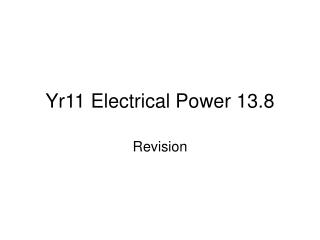DownloadDownload PresentationYr11 Electrical Power 13.8

# Yr11 Electrical Power 13.8

Download Presentation## Yr11 Electrical Power 13.8

- - - - - - - - - - - - - - - - - - - - - - - - - - - E N D - - - - - - - - - - - - - - - - - - - - - - - - - - -
##### Presentation Transcript

1. Yr11 Electrical Power 13.8 Revision

2. Water flows in a river Current = how much water flows in a certain time Current = the rate of flow of water

3. Charge flows in an electrical circuit Current = how much charge flows in a certain time Current = the rate of flow of charge

4. Charge is given electrical energy by the power supply The charge loses energy when it flows through components in the circuit. When it flows through a resistor it changes to heat energy.

5. The power of a device or component I how quickly it transfers energy Put these 4 devices in order of increasing power

6. 1. How much heat energy does a 3kW kettle produce in 2 minutes? P = 3,000W t = 120s So E.T. = P x t = 3,600,000J 2. An electric fire produces 500,000J of heat energy in 3 minutes. Calculate its power. 3. How long will it take a 2kW water heater to give 300,000J of heat energy to the water inside it?

7. A current of 0.5A flows through a 240V bulb. Calculate its power. • C = 0.5A V = 240V • P = V x C = 240 x 0.5 = 120W 2. What current flows through a 60W, 240V bedside lamp? 3. Which of these fuses should be used inside its plug? 1A 5A 10A 15A 30A

8. Higher Tier Only